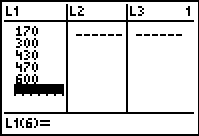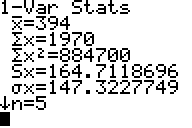# Knowledge Base

## Solution 12009: Calculating Variance on the TI-83 Plus Family of Graphing Calculators.

### How is variance calculated on the TI-83 Plus family of graphing calculators?

The TI-83 Plus family of graphing calculators calculates two types of standard deviation: the standard deviation of the sample and the population standard deviation. The population standard deviation, denoted by sx, divides the calculated values by n. The sample standard deviation, denoted by Sx, uses the value n-1 as the denominator.

The graphing calculators use the sample standard deviation Sx when calculating the variance (Sx2).

To find the variance using the population standard deviation, take the value of sx and raise it to the power of 2 (sx2).

Please Note: The sx variable will not have a value stored to it unless a 1-Var or 2-Var Stats analysis is performed first. Without a value stored to sx, calculating sx2 will result in an "UNDEFINED" error.

For example:

Using the data {170, 300, 430, 470, 600} find the variance.

1) Press [STAT] followed by [ENTER].
2) Enter in 170 and press [ENTER].
3) Follow this step for the remainder of data points.4) Press [2nd] followed by [QUIT].
5) Press [STAT] and scroll to the right.
6) Either press  or [ENTER].
7) Press [ENTER].8) Press [VARS].
9) Either press  or scroll down to option 5 and press [ENTER].
10) Either press  or scroll down to option 4 and press [ENTER].
11) Press [X²].
12) Press [ENTER].Please Note: The variance( command will find the sample variance which is the sample standard deviation of x raised to the power of 2.